# C Program to Print Star and Pyramid Patterns

In this article, you will learn and get code about how to print some famous patterns in C using stars and numbers, like the half-pyramid pattern and the full-pyramid pattern. A list of some famous patterns given in this article are:

## Pattern Programs in C

To print patterns of numbers and stars in C programming, you have to use two for loops, the outer for loop and the inner for loop. The outer for loop is responsible for rows, and the inner for loop is responsible for columns.

Here are one-by-one C programs to print different patterns:

### Print the half-pyramid using stars in C

This program prints a half-pyramid using a star pattern.

```#include<stdio.h>
#include<conio.h>
int main()
{
int i, j;
for(i=0; i<5; i++)
{
for(j=0; j<=i; j++)
printf("* ");
printf("\n");
}
getch();
return 0;
}```

As this program is written in the Code::Blocks IDE, here is the snapshot of the sample run: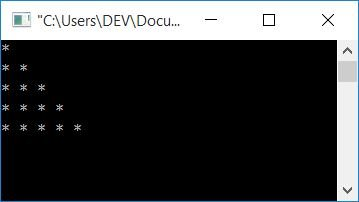#### Program Explained

1. Initialize any two integer variables, say i and j.
2. Here, variable i works for rows, and variable j works for columns.
3. It means that if you increment the value of variable i, the operation goes to the next row.
4. And if you increment the value of variable j, the operation goes to the next column.
5. Before moving on to the next row, make sure to break the line or use a line break statement `printf("\n");`
6. So here, initially, the value of variable i gets initialized with 0, and 0 is obviously less than 5, therefore the condition evaluates to true, then program flow goes inside the outer for loop.
7. The value of variable j gets initialized with 0, and it is checked whether the value of j (which is 0) is either less than or equal to the value of i (which is 0) or not.
8. The condition evaluates to true, therefore program flow goes inside the inner for loop, and one * (star) gets printed.
9. Again, the program flow goes to the third statement of the inner for loop, where the value of j gets incremented and becomes 1.
10. Again, the program flow goes to the second statement of the inner for loop and checks whether j<=i (1<=0) or not; this time, the condition evaluates to false.
11. So program flow does not go inside the inner for loop, but rather it goes to the third statement of the outer for loop.
12. There, the value of i gets incremented (like 0++), and the condition i<5 evaluates to true as 1<5 is true, then program flow again goes inside the outer for loop.
13. Now start from the 7th step (with the updated value of i) and process the code until the condition of the outer for loop evaluates to false.

### Print the star pattern in C

This program prints the star pattern in the following way:

• There is one star in the first row.
• There are three stars in the second row.
• In the third row, there are five stars.
• The fourth row has seven stars.
• The fifth row has nine stars.

One vertical line represents the first asterisk of each first column in each row. Let's take a look at the program for better understanding:

```#include<stdio.h>
#include<conio.h>
int main()
{
int i, j, k=1;
for(i=0; i<5; i++)
{
for(j=0; j<k; j++)
printf("* ");
k=k+2;
printf("\n");
}
getch();
return 0;
}```

When the above C program is compiled and executed, it will produce the following result: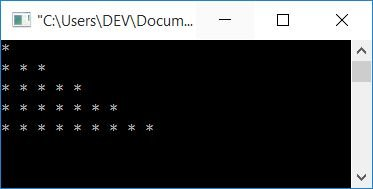#### Program Explained

• Here we have initialized one extra variable, say k, with 1 as its initial value.
• And instead of comparing j with i, we have compared it with k this time.
• Because 1, 3, 5, 7, and 9 stars must be printed in the first, second, third, fourth, and fifth rows,
• We have incremented the value of k by 2 each time after exiting from the inner for loop's statement.
• As a result, j (which initially holds 0) is compared to 1 first.
• When the program flow moves to the inner for loop after the outer one, j (which initially holds 0) is compared with 3. Initially, o<3 evaluates to true, so the program flow goes inside the inner for loop and the statement is executed, the value of j is incremented and becomes 1, compared with k (holds 3), so condition 1<3 evaluates to true, the program flow goes inside the inner for loop and the statement is executed, the value of j is incremented and becomes 2, compared with k or checks the condition j<k or (2<3) evaluates to true, the program flow goes inside the inner for loop once more, and when it executes its statement, the value of j is increased, and becomes 3, and the condition 3<3 now evaluates to false, so the program flow proceeds to the outer for loop's increment part and continues the program until the condition of the outer for loop evaluates to false.
• Each time the inner for loop runs, j (which initially holds 0) is compared to 5.
• At the fourth time, j gets compared with 7, each run of the inner for loop.
• At the fifth time, j gets compared with 9, each run of the inner for loop.
• For example, because the value of k is initially 1, the inner for loop's statement is only executed once the first time.
• The inner for loop's statement is executed three times the second time. Because its condition j<k evaluates to true three times, namely j<k (0<3), j<k (1<3), and j<k (2<3)
• In this way, 1, 3, 5, 7, and 9 stars get printed on the first, second, third, fourth, and fifth rows, as shown in the snapshot given above.

The following program prints the first half of a pyramid with a star pattern in the following manner:

• In the first row, there are 8 spaces and 1 star.
• In the second row, there are 6 spaces and 2 stars.
• In the third row, there are four spaces and three stars.
• In the fourth row, there are two spaces and four stars.
• In the fifth row, there is one space and five stars.

After each asterisk, add a space. Let's take a look at the program:

```#include<stdio.h>
#include<conio.h>
int main()
{
int i, j, space=8;
for(i=0; i<5; i++)
{
for(j=0; j<space; j++)
printf(" ");
space = space-2;
for(j=0; j<=i; j++)
printf("* ");
printf("\n");
}
getch();
return 0;
}```

Here is a snapshot of the sample run:Before printing a star on each row, first print spaces. That is 8, 6, 4, 2, and 0 space on the first, second, third, fourth, and fifth rows. and 1, 2, 3, 4, and 5 stars (after spaces) on each row.

The following program prints the star pattern in the following ways:

• 16 spaces and 1 star
• 12 spaces and 3 stars
• 8 spaces and 5 stars
• 4 spaces and 7 stars
• 0 space and 9 stars

After each asterisk, add a space. Let's take a look at the program given here:

```#include<stdio.h>
#include<conio.h>
int main()
{
int i, j, space=16, k=1;
for(i=0; i<5; i++)
{
for(j=0; j<space; j++)
printf(" ");
space = space-4;
for(j=0; j<k; j++)
printf("* ");
k = k+2;
printf("\n");
}
getch();
return 0;
}```

A snapshot of the sample run of the above program is given here: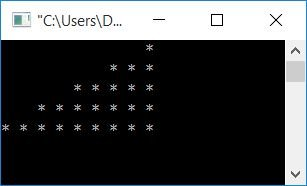This program is a little bit similar to the above one. Except in this case, we have to print 16 spaces at first and then decrease them by 4 each time. And print 1 star at first, then increment the value of the star by 2 each time you go to the next row.

### Print the half pyramid using natural numbers in C

This program prints a half-pyramid using natural numbers:

```#include<stdio.h>
#include<conio.h>
int main()
{
int i, j, num=1;
for(i=0; i<5; i++)
{
for(j=0; j<=i; j++)
{
printf("%d ", num);
num++;
}
printf("\n");
}
getch();
return 0;
}```

Here is the screenshot of the sample run: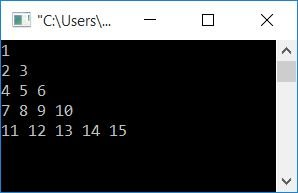As you can see from the above program, the code for both the loops is the same as the very first program in this article, except that here we have used another variable num so that after initializing it with 1, we have incremented it by 1 each time to print a natural number that starts at 1. Without regard for rows and columns in this case.

### Print the half pyramid using natural numbers on each row in C

This program also prints a half-pyramid using natural numbers, but not as in the previous program. This program prints patterns in the following ways:

• 1 on the first row
• on the second row, 1 2
• on the third row, 1 2 3
• on the fourth row, 1 2 3 4
• on the fifth row, 1 2 3 4 5

As you can see, we must initialize the value of num with 1 each time before entering the inner for loop, which is in charge of columns. To print a natural number, begin at 1 on each and every row. Here is the program:

```#include<stdio.h>
#include<conio.h>
int main()
{
int i, j, num=1;
for(i=0; i<5; i++)
{
num = 1;
for(j=0; j<=i; j++)
{
printf("%d ", num);
num++;
}
printf("\n");
}
getch();
return 0;
}```

Let's take a look at the sample output of the above program as given in the snapshot here: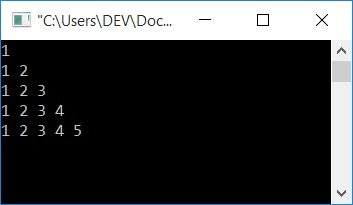### Print the full pyramid using stars in C

This program prints a pure pyramid pattern using a star. And here the user decides the height of the pyramid at runtime. That is, it asks the user to enter the number of rows to print a pyramid of stars, as instructed by the C program:

```#include <stdio.h>
#include<conio.h>
int main()
{
int i, space, rownum, k=0;
printf("Enter the number of rows : ");
scanf("%d", &rownum);
for(i=1; i<=rownum; i++)
{
for(space=1; space<=(rownum-i); space++)
printf("  ");
while(k!=(2*i-1))
{
printf("* ");
k++;
}
k=0;
printf("\n");
}
getch();
return 0;
}```

When you execute the above program at your end, then here is a snapshot of the initial output you will see on your output screen: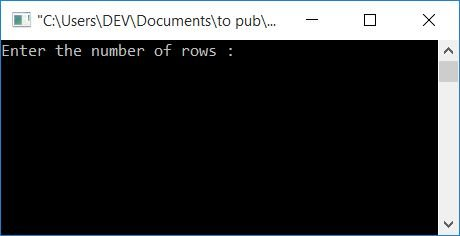Now enter any number of rows, say 8, and press the ENTER key to see the output as shown in the snapshot given here: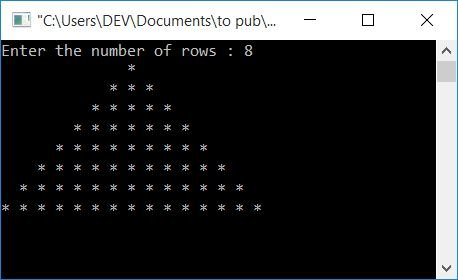#### The same program in different languages

C Quiz

« Previous Program Next Program »